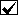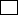# Cardinal and Ordinal Numbers

Q1 - OneCardinal numberOrdinal number
Q2 - ThirdCardinal numberOrdinal number
Q3 - NoughtCardinal numberOrdinal number
Q4 - FirstCardinal numberOrdinal number
Q5 - We use ____ numbers with dates.cardinalordinal
Q6 - We use ____ numbers with names of kings and queens.cardinalordinal
Q7 - We use ____ numbers for hotel rooms.cardinalordinal
Q8 - We use ____ for floors of a building.cardinalordinal
Q9 - We use ____ numbers for a person's age.cardinalordinal
Q10 - We use ____ numbers with names of popes.cardinalordinal